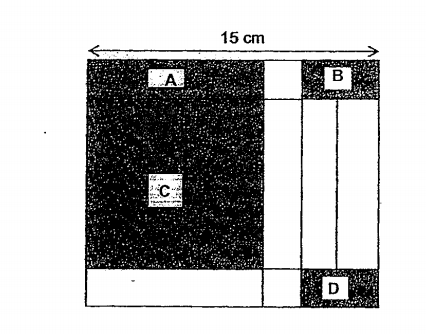# Question 1 of 50

The figure below shows a piece of cardboard of length 15cm. When the shaded rectangles A, B, D and square C are cut out, the remaining parts form the net of cuboid. Given that the area of A is 10cm$^2$ and the area of C is 81cm$^2$. Find the volume of the cuboid.A
25cm$^3$
B
28cm$^3$
C
32cm$^3$
D
36cm$^3$
E
None of the above